Courses

# ICAI Notes 4.2 - Determination of Prices CA Foundation Notes | EduRev

## CA Foundation : ICAI Notes 4.2 - Determination of Prices CA Foundation Notes | EduRev

The document ICAI Notes 4.2 - Determination of Prices CA Foundation Notes | EduRev is a part of the CA Foundation Course Economics for CA CPT.
All you need of CA Foundation at this link: CA Foundation

Learning Objectives

 how prices are generally determined.
 how changes in demand and supply affect prices and quantities demanded and supplied.

2.0 INTRODUCTION

Prices of goods express their exchange value. These are also used for expressing the value of various services rendered by different factors of production such as land, labour, capital and organization. These values respectively are, rent, wages, interest and profit. Therefore, the concept of price, especially the process of price determination, is of vital importance in Economics.

It is to be noted that generally it is the interaction between demand and supply that determines the price but sometimes Government intervenes and determines the price either fully or partially. For example, the Government of India fixes up prices of petrol, diesel, kerosene, coal, fertilizers, etc. which are critical inputs. It also fixes up procurement prices of wheat, rice, sugarcane, etc. in order to protect the interests of both producers and consumers. While determining these prices, the Government takes into account factors like cost of inputs, risks for business, nature of the product etc.

2.1 DETERMINATION OF PRICES - A GENERAL VIEW

In an open competitive market it is the interaction between demand and supply that tends to determine price and quantity. This can be shown by bringing together demand and supply. Combining the tables of demand and supply (on page 33 and 66 respectively) of Chapter-2, we have the following schedule :

Table – 2 : Determination of Price

 S.No Price(Rs.) Demand(Units) Supply (Units) 1 1 60 5 2 2 35 35 3 3 20 45 4 4 15 55 5 5 10 65

When we plot the above points on a single graph with price on Y-axis and quantity demanded and supplied on X-axis, we get a figure like this :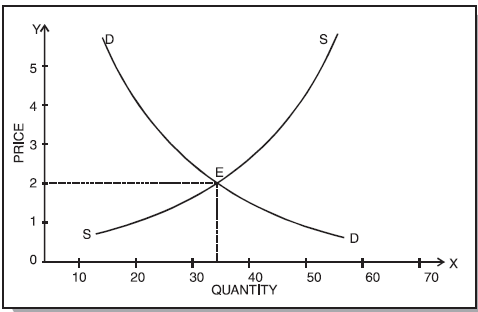Fig. 1 : Determination of Equilibrium Price

It is easy to see which will be the market price of the article. It cannot be Re. 1, for at that price there would be 60 units in demand, but only 5 units on offer. Competition among buyers would force the price up. On the other hand, it cannot Rs. 5, for at that price there would be 65 units on offer for sale but only 10 units in demand. Competition among sellers would force the price down. At Rs. 2, demand and supply are equal (35 units) and the market price will tend to settle at this figure. This is equilibrium price and quantity – the point at which price and output will tend to stay. Once this point is reached we will have stable equilibrium. It should be noted that it would be stable only if other things were equal.

2.2 CHANGES IN DEMAND AND SUPPLY

The facts of real world, however, are such that other things (like income, tastes and preferences, population, etc.) always change causing changes in the demand and supply. The four main changes in demand and supply are :

(i) An increase (shift to the right) in demand;

(ii) A decrease (shift to the left) in demand;

(iii) An increase (shift to the right) in supply;

(iv) A decrease (shift to the left) in supply.

We will consider each of the above changes one by one.

(i) An increase in demand : In figure 2, the original demand curve is DD and supply curve is SS. At equilibrium price OP, demand and supply are equal to OQ.

Now suppose the money income of the consumer increases, the demand curve will shift to D1D1 and the supply curve will remain same. We will see that on the new demand curve D1Dat OP price demand increases to OQ2 while supply remains the same i.e. OQ. Since supply is short of the demand, price will go up to OP1. With the higher price supply will also shoot up and new equilibrium between the demand and supply will be reached. At this equilibrium point, OP1 is price and OQ1 is the quantity which is demanded and supplied.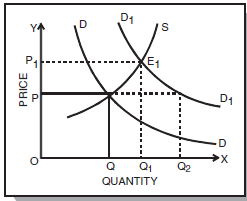Fig. 2 : Increase in Demand, causing an increase in equilibrium price and quantity

Thus, we see that as a result of an increase in demand, there is an increase in equilibrium price, as a result of which the quantity sold and purchased also increases.

(ii) Decrease in Demand : Opposite will happen when the demand falls as a result of a fall in income, while the supply remaining the same. The demand curve will shift to the left and become D1D1 while the supply curve remaining as it is. With the new demand curve D1D1 at original price OP, OQis demanded and OQ is supplied. As the supply exceeds demand, price will go down and quantity demanded will go up. A new equilibrium price OP1 will be settled in the market where demand OQ1 will be equal to supply OQ1.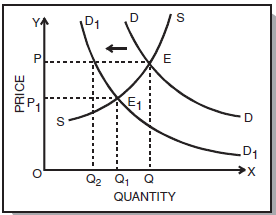Fig. 3 : Decrease in Demand resulting in a decrease in price and quantity demanded

Thus with a decrease in demand, there is a decrease in the equilibrium price and quantity demanded and supplied.

(iii) Increase in Supply : Let us now assume that demand does not change, but there is an increase in supply say, because of improved technology.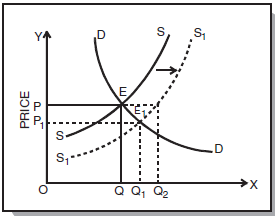Fig. 4 : Increase in supply, resulting in decrease in equilibrium price and increase in quantity supplied

The supply curve SS will shift to the right and become S1S1. At the original equilibrium price OP, OQ is demanded and OQ2 is supplied (with new supply curve). Since the supply is greater than the demand, the equilibrium price will go down and become OP1 at which OQ1 will be demanded and supplied.

Thus, as a result of an increase in supply the equilibrium price will go down and the quantity demanded will go up.

(iv) Decrease in Supply : If because of some reason, there is a decrease in the supply we will find that equilibrium price will go up but the amount sold and purchased will go down as shown in figure 5 :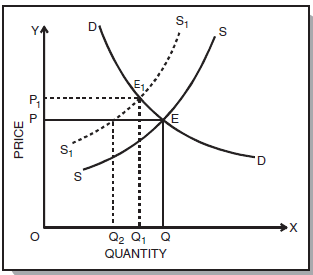Fig. 5 : Decrease in supply causing an increase in the equilibrium price and a fall in quantity demanded

2.3 SIMULTANEOUS CHANGES IN DEMAND AND SUPPLY

Till now, we were considering the effect of change either in demand or in supply on the equilibrium price and the quantity sold and purchased. There may be cases in which both the supply and demand change at the same time. During a war, for example, shortage of goods will often decrease supply while full employment causes high total wage payments which increase demand.

We may discuss the changes in both demand and supply with the help of diagrams as follows :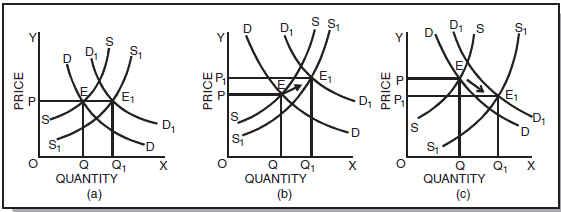Fig.6 : Simultaneous Change in Demand and Supply

Fig. 6 shows simultaneous change in demand and supply and its effects on the equilibrium price. In the figure, the original demand curve DD and the supply curve SS meet at E at which OP is the equilibrium price OQ is the quantity bought and sold.

Fig. 6 (a), shows that increase in demand is equal to increase in supply. The new demand curve D1D1 and S1S1 meet at E1. The new equilibrium price is equal to the old equilibrium price (OP).

Fig. 6 (b), shows that increase in demand is more than increase in supply. Hence, the new equilibrium price OP1 is higher than the old equilibrium price OP. Opposite will happen i.e. the equilibrium price will go down if there is a simultaneous fall in the demand and supply and the fall in demand is more than the fall in supply.

Fig. 6 (c), shows that supply increases in a greater proportion than demand. The new equilibrium price will be less than the original equilibrium price. Conversely, if the fall in the supply is more than proportionate to the fall in the demand, the equilibrium price will go up.

SUMMARY

The price of a product depends upon (i) its demand and (ii) its supply. Demand for a product in turn depends upon utility it provides to consumers and the supply on the cost of producing it. Equilibrium price is determined at a point where demand is equal to supply. Here, all other things are supposed to be equal.

However, we seldom get a stable equilibrium. Conditions underlying demand and supply keep on changing and the demand and supply curves keep on shifting giving rise to a new equilibrium price. Supply remaining same, if demand increases, equilibrium price will move up and if demand decreases, the equilibrium price will move down. Demand remaining same, if the supply increases the equilibrium price will decline and vice-versa.

There can be simultaneous change in both demand and supply and the equilibrium price will change according to the proportionate change in demand and supply.

,

,

,

,

,

,

,

,

,

,

,

,

,

,

,

,

,

,

,

,

,

;